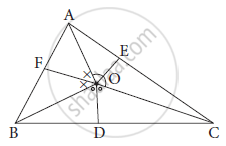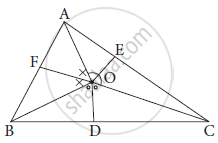Advertisement Remove all ads

# O is Any Point in the Interior of δAbc. Bisectors of ∠Aob, ∠Boc and ∠Aoc Intersect Side Ab, Side Bc, Side Ac Inf, D and E Respectively.Prove Thatbf × Ae × Cd = Af × Ce × Bd - GeometryO is any point in the interior of ΔABC. Bisectors of ∠AOB, ∠BOC and ∠AOC intersect side AB, side BC, side AC in
F, D and E respectively.
Prove that
BF × AE × CD = AF × CE × BD

Advertisement Remove all ads

#### SolutionIn Δ AOB, OF is bisector of ∠ AOB
∴(OA)/(OB) = (AF)/(BF) ....... (1) (by angle bisector theoerm)

In Δ BOC, OD is bisector of angle ∠ BOC .
∴(OB)/(OC) =( BD)/(CD) ....... (2)(by angle bisector theoerm)
In Δ AOC , OE is bisector of angle∠ AOC.

∴( OC)/(OA) = (CE)/(AE) ....... (3)(by angle bisector theoerm)
∴ (OA)/(OB) ×( OB)/(OC) × (OC)/(OA) = (AF)/(BF) ×( BD)/(CD) × (CE)/(AE) from (1), (2) and (3)

therefore(OAxxOCxxOB)/(OBxxOAxxOC) = (AFxxBFxxCE)/(BDxxAExxCD)

 therefore1 = (AFxxCExxBD)/(BFxxAExxCD)

∴ BF × AE × CD = AF × CE × BD

Is there an error in this question or solution?
Advertisement Remove all ads
Advertisement Remove all ads
Share
Notifications

View all notifications

Forgot password?